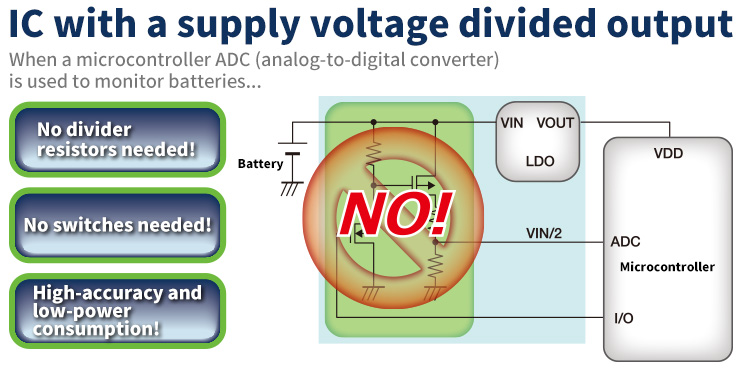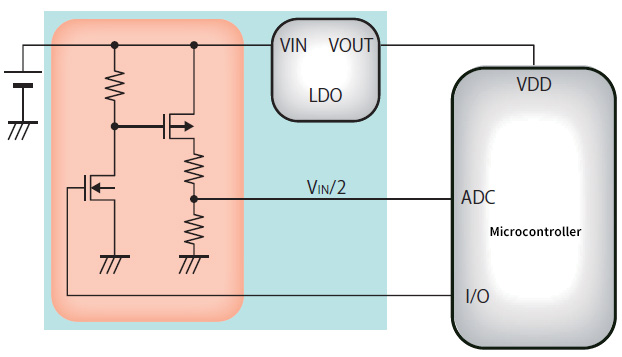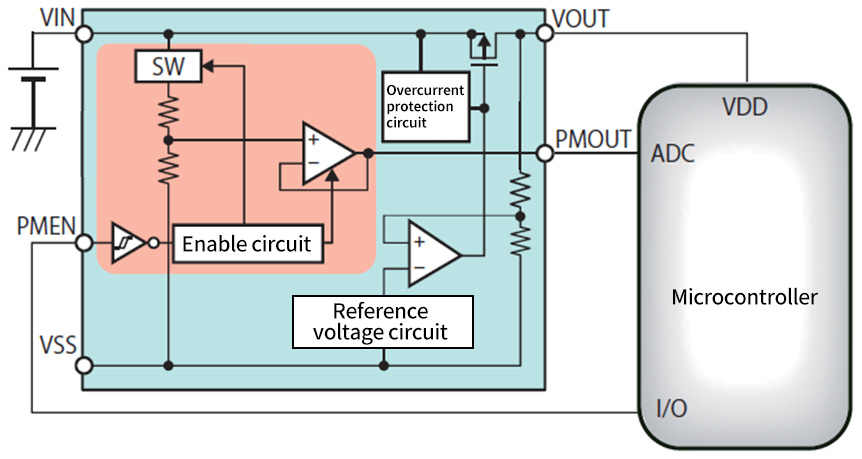# Simple battery monitoring! IC with a “supply voltage divided output”!### No divider resistors needed! No switches needed! No calculations needed!”– Simple, high-accuracy battery monitoring! “Supply voltage divided output” –

A product that runs on battery power needs a battery monitor function to keep track of how much power is left. This is normally done using a divider resistor that divides the power voltage and inputs it to a microcontroller ADC. A divider resistor is used to divide the power voltage to prevent the monitored battery voltage from exceeding the withstand voltage of the microcontroller that is used. To conserve battery power, the current flowing through the divider resistor is controlled by a transistor. (The red portion in the figure below)
As more parts are added to implement the required functions, the selection of parts becomes more complex.In calculating the resistance of the divider resistor, it is necessary to consider the internal impedance of the connected ADC. If this impedance is omitted when determining the resistance, the required voltage will not be input to the ADC making it impossible to properly monitor the battery. The transistor ON resistance that functions as a switch may also impact output accuracy depending on the resistance value.
An increase in the number of components may also make it necessary to increase the size of the mounted board. An IC with a supply voltage divided output can be used to solve the above problems of “complexity in selecting parts,” “increase in the number of parts” and “increase in the size of the mounted board.”

An IC with a supply voltage divided output is an IC that has a function for dividing battery voltage and output a voltage that can be input to an ADC. (The red portion in the figure below)An IC with a supply voltage divided output is equipped not only with a divider resistor for dividing voltage, but also integrates an output analog buffer and an output ON/OFF switch.
The divided voltage is output via the analog output buffer so the internal impedance of the ADC never becomes an issue.
The integration of the switch and switching the output ON and OFF make it possible to reduce current consumption. The IC with a supply voltage divided output takes care of resistance calculation and selection problems.
Since the divider resistor, the output analog buffer and the output ON/OFF switch are integrated, there is not an excessive number of parts to inspect and the mounted board can be kept compact.

ABLIC both designs and manufactures the power supply IC with the supply voltage divided output that solves the problems that occur when dividing the power supply voltage using a divider resistor.ABLIC was presented with the Award for Excellence in the Semiconductor device category in the “Semiconductor of the Year 2018” sponsored by Electronic Device Industry News for the S-85S1P Series of ultra-high-efficiency buck converters with a supply voltage divided output.